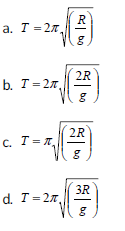# JEE Main Sample Paper 2015 Set E

JEE Main Sample Paper 2015 Set E. Please see the link below for the sample paper for the upcoming JEE (Main) examination which is conducted by CBSE for candidates to get admission into various engineering colleges like NITs and IIITs and other affiliated colleges all over India. Candidates are suggested to download the sample paper/ model test paper and practice the questions for the upcoming JEE (Main) examinations.

PHYSICS

Q1. Ten litre of water per second is lifted from well through 20 m and delivered with a velocity of 10m/s, then the power of the motor is:

a. 1.5 kW
b. 2.5 kW
c. 3.5 kW
d. 4.5 kW

Q2. A ring of mass m and radius R is pivoted at a point O on its periphery. It is free to rotate about an axis perpendicular to its plane. What is the period of ring?Q3. Output W is given by :Q4. A car is moving rectilinearly on a horizontal path with acceleration α0. A person sitting inside the car observes that an insect S is crawling up the screen with an acceleration α. If θ is the inclination of the screen with the horizontal, acceleration of the insect:

a. Parallel to the screen is α + α0 cos θ
b. Along the horizontal is α0 + α cos θ
c. Perpendicular to the screen is α0 sin θ
d. Perpendicular to the screen is α0 tan θ

Q5. Bernoulli’s equation is applicable to points:

a. In a steadily flowing liquid
b. In a stream line
c. In a straight line perpendicular to a stream line
d. In any viscous liquid.

Q6. If vs, vx and vm are the speeds of gamma rays ,X-rays and microwaves respectively in the vacuum then :

a)vs> vx >vm
b)vs< vx< vm
c)vs> vx< vm
d)vs = vx = vm

Q7. If the angular momentum of a body increases by 40% its kinetic energy of rotation increases by:

a. 80%
b. 20%
c. 96%
d. None of these

Q8. When two coherent monochromatic light beams of intensities I and 4I are superimposed, what are the maximum and minimum possible intensities in the resulting beams?

a) 9I and I
b) 9I and 3I
c) 5I and I
d) 9I and 3I

Refer to link below JEE Main Sample Paper 2015 Set E